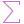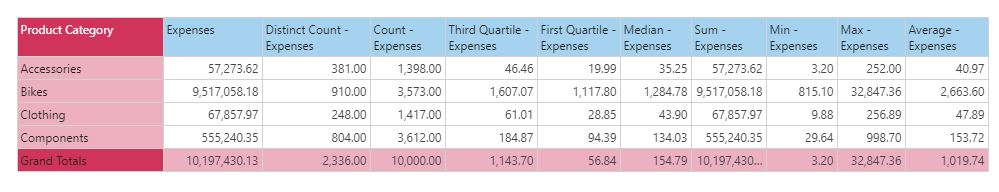#Median (PQL)

Executes median aggregation on a column of data from the model. The column needs to have numerical data for it to be functional.

• Returned Output: Numeric
• Library: PQL \ Semantic \ Aggregate
• Version: 2018.00.000
• Compatibility: Pyramid Query Language (PQL) data sources

#### Syntax

Median( <Measure> )

* Click on the function's arguments above for more details on the input values.

• The "measure" column needs to be the name of the column in the model [table].[column name] or it can be an existing measure in the model [measures].[column name].
• For details on how to employ and use this function see the granular calculation overview.

NOTE: The median/quartile function is not supported by all data sources.

##### Different Function types
• This function is like the SQL median function; calculating the median, or 50th percentile, of a column of data in your query or model .
• If you wish to find the median for the elements in a specified SET or LIST from a hierarchy, use this semantic function.
• To use a simple median mathematical operation on a list of numbers, use the Common library function.

#### Examples

To create a new measure that is the median of expenses transactions in the model, using the sample demo model:

Median( [data].[Expenses] )

or:

Median( [measures].[data Expenses] )

The mechanics of this function are shown below. According to the grid, the median or 50th percentile of all expense transactions across all 'Accessory' transactions is 35.25.